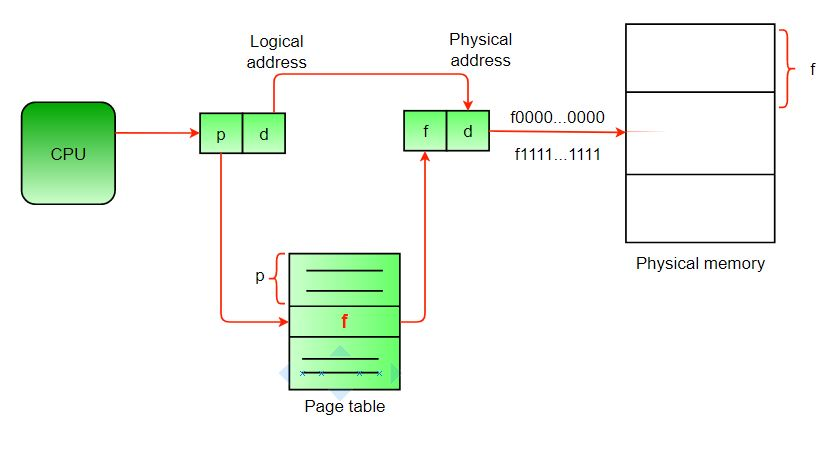# Operating Systems | Set 2

Following questions have been asked in GATE CS exam.

1. Consider a machine with 64 MB physical memory and a 32-bit virtual address space. If the page size is 4KB, what is the approximate size of the page table? (GATE 2001)
(a) 16 MB
(b) 8 MB
(c) 2 MB
(d) 24 MB

Explanation:
A page entry is used to get address of physical memory. Here we assume that single level of Paging is happening. So the resulting page table will contain entries for all the pages of the Virtual address space.

```Number of entries in page table =
```

Using above formula we can say that there will be 2^(32-12) = 2^20 entries in page table.
No. of bits required to address the 64MB Physical memory = 26.
So there will be 2^(26-12) = 2^14 page frames in the physical memory. And page table needs to store the address of all these 2^14 page frames. Therefore, each page table entry will contain 14 bits address of the page frame and 1 bit for valid-invalid bit.
Since memory is byte addressable. So we take that each page table entry is 16 bits i.e. 2 bytes long.

```Size of page table =
(total number of page table entries) *(size of a page table entry)
= (2^20 *2) = 2MB
```

For the clarity of the concept, please see the following figure. As per our question, here p = 20, d = 12 and f = 14.2. Consider Peterson’s algorithm for mutual exclusion between two concurrent processes i and j. The program executed by process is shown below.

```   repeat
flag [i] = true;
turn = j;
while ( P ) do no-op;
Enter critical section, perform actions, then exit critical
section
flag [ i ] = false;
Perform other non-critical section actions.
until false; ```

For the program to guarantee mutual exclusion, the predicate P in the while loop should be (GATE 2001)
a) flag [j] = true and turn = i
b) flag [j] = true and turn = j
c) flag [i] = true and turn = j
d) flag [i] = true and turn = i

Basically, Peterson’s algorithm provides guaranteed mutual exclusion by using the two following constructs – flag[] and turn. flag[] controls that the willingness of a process to be entered in critical section. While turn controls the process that is allowed to be entered in critical section. So by replacing P with the following,

flag [j] = true and turn = j

process i will not enter critical section if process j wants to enter critical section and it is process j’s turn to enter critical section. The same concept can be extended for more than two processes. For details, refer the following.
References:
http://en.wikipedia.org/wiki/Peterson%27s_algorithm

3 More than one word are put in one cache block to (GATE 2001)

(a) exploit the temporal locality of reference in a program
(b) exploit the spatial locality of reference in a program
(c) reduce the miss penalty
(d) none of the above

Temporal locality refers to the reuse of specific data and/or resources within relatively small time durations. Spatial locality refers to the use of data elements within relatively close storage locations.
To exploit the spatial locality, more than one word are put into cache block.
References:
http://en.wikipedia.org/wiki/Locality_of_reference

4. Which of the following statements is false? (GATE 2001)

a) Virtual memory implements the translation of a program’s address space into physical memory address space
b) Virtual memory allows each program to exceed the size of the primary memory
c) Virtual memory increases the degree of multiprogramming
d) Virtual memory reduces the context switching overhead

In a system with virtual memory context switch includes extra overhead in switching of address spaces.
References:

5. Consider a set of n tasks with known runtimes r1, r2, … rn to be run on a uniprocessor machine. Which of the following processor scheduling algorithms will result in the maximum throughput? (GATE 2001)

(a) Round-Robin
(b) Shortest-Job-First
(c) Highest-Response-Ratio-Next
(d) First-Come-First-Served